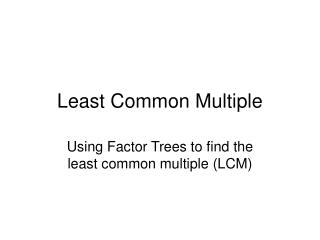DownloadDownload PresentationLeast Common Multiple

# Least Common Multiple

Download Presentation## Least Common Multiple

- - - - - - - - - - - - - - - - - - - - - - - - - - - E N D - - - - - - - - - - - - - - - - - - - - - - - - - - -
##### Presentation Transcript

1. Least Common Multiple Using Factor Trees to find the least common multiple (LCM)

2. Least Common Multiple • The least common multiple of two (or more) numbers is the smallest number that can be divided by both (or all) the numbers. • Example the LCM of 4 and 7 is 4x7 = 28

3. Finding the LCM • To find the LCM we can list all the multiples of each number and find the first one that appears in each list • Eg LCM 24 and 36 • 24, 48, 72, 96, 120 . . . • 36, 72, 108, 144 . . . • So the LCM of 24 and 36 is 72

4. But, if you have big/nasty numbers • This is takes ages • Easy to make a mistake

5. LCM – Using Factor Trees

6. Find the LCM of 45 and 72 • First write each number as a product of its primes • Find the Highest Common Factor by identifying the primes which appear in both numbers • Find the Lowest Common Multiple by multiplying the Highest Common Factor by all the factors that are leftover. HELP!!

7. 45 72 1. Product of Primes 72 45 2 36 5 9 2 18 3 3 2 9 3 x 3 x 5 = 45 32 x 5 = 45 2 x 2 x 2 x 3 x 3 = 72 23 x 32 = 72 3 3

8. 45 72 2. Highest Common Factor 72 45 2 36 5 9 2 18 3 3 2 9 3 3 HCF = 3 x 3 = 9

9. 45 72 72 45 2 36 5 9 2 18 3 3 2 9 3 3 3. LCM = HCF x Leftovers HCF = 3 x 3 = 9 Leftovers = 5 x 23 = 40 LCM =9x40= 360

10. 18 and 30 27 and 36 14 and 35 15 and 55 24 and 56 35 and 42 56 and 100 108 and 126 90 108 70 165 168 210 1400 756 Find the HCF and then LCM of the following: